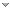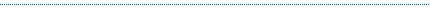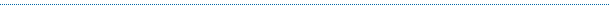About QweasRSS Feeds     BBS Forum Make Qweas.com My Home Page     Bookmark this page Register     Login     Help     Send Feedback
 All software Windows Mac Linux Mobile Games Screensavers Source Code Webware
Windows   Mac   Linux   Mobile   Games   Screensavers
 Audio/Video Business Communication Desktop Develop Education Games Graphic Home Network Security Servers System Web Computer , Dictionaries , Geography , Kids , Languages , Mathematics , Other , Reference Tools , Science , Teaching & Training Tools
 Recommends 4Media DPG ConverteriPod 2 iPodSuper Mp3 RecorderABC Amber Thunderbird ConverterAllok Video SplitterDRM Converter 3 for MacVentaFax & VoiceJoboshare DVD CopyiSofter DVD to MP4 Converter321Soft Screen Video RecorderInsaniquarium CD2000th HellFire screensaver

 Related Reviews - more mathematics softwaremathematics software for kidsmathematics teaching softwaremathematical gamesmathematics imagingmathematics analysiscomputer securitycomputer gamescomputer monitoringcomputer privacy protectioncomputer backupcomputer repaircomputer performancecomputer protectioncomputer configurationdictionary softwareenglish dictionarychinese dictionaryother language dictionarydictionary design
Home > Education > Mathematics > Visual Fractal > Publisher

# Visual Fractal Publisher

## Fractal software to create Mandelbrot fractal, Julia fractal etc.

Publisher: GraphNow Software

Website: http://www.graphnow.comGraphNow Software develops: Visual Complex : Graph software to graph complex functions ; Visual Probability : Distribution computing and graphing. ; Visual Calculus : Calculus grapher for calculus teaching. ; Visual Fractal : Create Mandelbrot fractal, Julia fractal etc ; Visual Optim : Implement linear and nonlinear programming. ...# Visual Complex

Visual Complex is a graph software to create graph of complex function. 3D function graphs and 2D color maps can be created with this grapher. It is a graphing calculator to create a graph of complex function.# Visual Optim

Visual Optim is a math program for one dimension searching, linear programming, unconstrained nonlinear programming and constrained nonlinear programming. Visual Optim features include: One dimensional searching, Linear programming, and much more...# Visual Calculus

Visual Calculus is an easy-to-use calculus grapher for Graphing limit, derivative function, integral, 3D vector, series, ode etc. Visual Calculus can create 2D, 2.5D and 3D function graphs, animations and table graphs.# Visual Stats

Visual Probability is a software to compute and graph probability density function values, cumulative distribution function values, quantile values, means and variances of ten more distributions. visualizaton.# Visual Matrix

Visual Matrix is a matrix calculator to implement matrix operations and matrix decompositions. The features are including: Basic matrix operations: +, -, .*, *, ', determinant, etc.; Matrix decompositions: LU decomposition, QR decomposition.# Visual Fitting

Math tool for linear, nonlinear curve fitting and surface fitting, curve and surface can be created. It can implement curve fitting and graphing of linear and nonlinear models, can implement surface fitting and graphing of any binary function models.# Visual Fractal

Visual Fractal is an interesting grapher to create a graph of fractal. With this interesting fractal software, you can use Newton's method to solve a complex equation and show the fractal graph in the plot area# Visual Probability

Visual Probability is a software to compute and graph probability density function values, cumulative distribution function values, quantile values, means and variances of ten more distributions. visualizaton.

TAGS OF VISUAL FRACTALSite Map | Sort by Letters | Submit Software | Popular Downloads | Editor Picks | New Releases : Mac , Freeware | Updates : Mac , Freeware